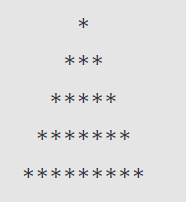Related Tags

javascript
nested loops
pyramid pattern
communitycreator

# How to print a pyramid pattern using JavaScript

Ayush Trivedi

### Overview

In this shot, we will learn to solve a common coding problem pattern. We will print a star pyramid pattern using JavaScript.

### Approach

We will use the following three for loops:

• One is the outer loop for rows of the pyramid.

• The other two are nested loops, where the first inner loop will print the number of spaces (' ') and the second will print the number of stars (*).

### Desired output

We will print the below pattern:### Code

Let’s see the program to create a pyramid pattern:

let n = 5;
// External loop
for (let i = 1; i <= n; i++) {
// printing spaces
for (let j = 1; j <= n - i; j++) {
process.stdout.write(' ')
}
// printing stars
for (let k = 0; k < 2 * i - 1; k++) {
process.stdout.write('*')
}
console.log();
}

### Explanation

• Line 1: We initialize a variable n with an integer representing the total number of rows that make up the pyramid. We can also consider this as the height of the pyramid.

• Line 3: We have the first for loop that will iterate over the total number of rows (5, in the example above).

• Lines 5 to 7: We have the first nested for loop to print spaces n-i times, where i is the current iteration of the outer loop. For example, if i= 3, we will print 2 spaces in the third row using process.stdout.write(' ').

• Lines 9 to 11: We have the second nested for loop to print stars 2 * i - 1 times, where i is the current iteration of outer loop. For example, if i= 3, we will print 5 stars in the third row using process.stdout.write('*').

• Line 12: We used console.log() with null, as it will change to a new line. We can also use process.stdout.write('\n') to change the line.

### Conclusion

We completed the task to print *'s in a form that looks like a pyramid. However, this shot shows only one way to do this. There can be several algorithms to achieve the same result.

RELATED TAGS

javascript
nested loops
pyramid pattern
communitycreator

CONTRIBUTOR

Ayush Trivedi
RELATED COURSES

View all Courses

Keep Exploring

Learn in-demand tech skills in half the time